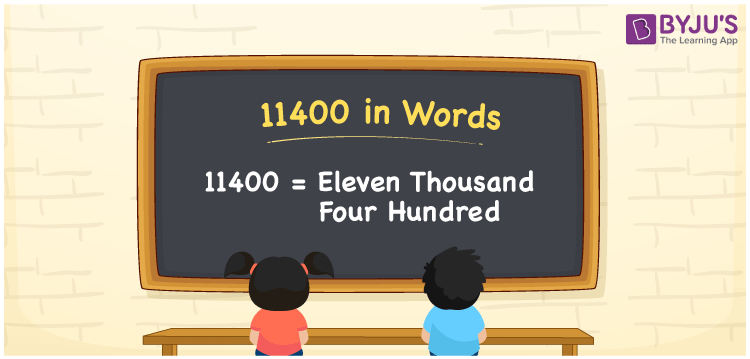# 11400 in words

11400 in words is written as Eleven Thousand Four Hundred. In 11400, 1 has a place value of ten thousand, second 1 has a place value of thousand and 4 has a place value of Hundred. The article on Place Value gives more information. The number 11400 is used in expressions that relate to money, distance, length, count and many more. For example, “Eleven Thousand Four Hundred Rupees is the amount I drew from the bank.”

 11400 in words Eleven Thousand Four Hundred Eleven Thousand Four Hundred in Numerical Form 11400

## 11400 in English Words## How to Write 11400 in Words?

We can convert 11400 to words using a place value chart. The number 11400 has 5 digits, so let’s make a chart that shows the place value up to 5 digits.

 Ten thousands Thousands Hundreds Tens Ones 1 1 4 0 0

Thus, we can write the expanded form as:

1 × Ten thousand + 1 × Thousand + 4 × Hundred + 0 × Ten + 0 × One

= 1 × 10000 + 1 × 1000 + 4 × 100 + 0 × 10 + 0 × 1

= 11400

= Eleven Thousand Four Hundred.

11400 is the natural number that is succeeded by 11399 and preceded by 11401.

11400 in words – Eleven Thousand Four Hundred.

Is 11400 an odd number? – No.

Is 11400 an even number? – Yes.

Is 11400 a perfect square number? – No.

Is 11400 a perfect cube number? – No.

Is 11400 a prime number? – No.

Is 11400 a composite number? – Yes.

## Solved Example

1. Write the number 11400 in expanded form

Solution: 1 x 10000 + 1 x 1000 + 4 x 100 + 0 x 10 + 0 x 1

Or Just 1 x 10000 + 1 x 1000 + 4 x 100

We can write 11400 =10000 + 1000 + 400 + 0 + 0

= 1 x 10000 + 1 x 1000 + 4 x 100 + 0 x 10 + 0 x 1

## Frequently Asked Questions on 11400 in words

Q1

### How to write 11400 in words?

11400 in words is written as Eleven Thousand Four Hundred.
Q2

### State True or False. 11400 is divisible by 4?

True. 11400 is divisible by 4.
Q3

### Is 11400 divisible by 10?

Yes. 11400 is divisible by 10.
Test your Knowledge on 11400 in Words